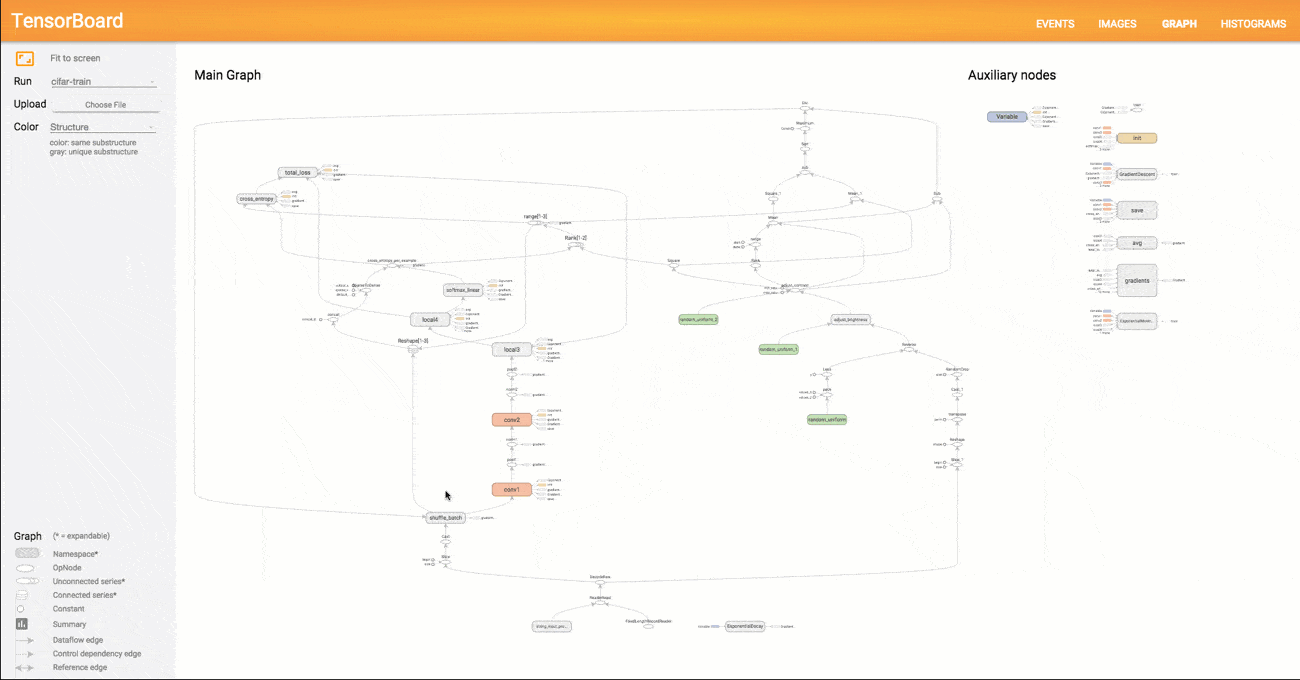# Chapter. Graph¶

TensorFlow uses a dataflow graph to represent your computation in terms of the dependencies between individual operations. A graph defines the computation. It doesn’t compute anything, it doesn’t hold any values, it just defines the operations that you specified in your code.

## Defining the Graph¶

We define a graph with a variable and three operations: `variable` returns the current value of our variable. `initialize` assigns the initial value of 31 to that variable. `assign` assigns the new value of 12 to that variable.

```with<Graph>(tf.Graph().as_default(), graph =>
{
var variable = tf.Variable(31, name: "tree");
tf.global_variables_initializer();
variable.assign(12);
});
```

TF.NET simulate a `with` syntax to manage the Graph lifecycle which will be disposed when the graph instance is no long need. The graph is also what the sessions in the next chapter use when not manually specifying a graph because use invoked the `as_default()`.

A typical graph is looks like below:image

## Save Model¶

Saving the model means saving all the values of the parameters and the graph.

```saver = tf.train.Saver()
saver.save(sess,'./tensorflowModel.ckpt')
```

After saving the model there will be four files:

• tensorflowModel.ckpt.meta:
• tensorflowModel.ckpt.data-00000-of-00001:
• tensorflowModel.ckpt.index
• checkpoint

We also created a protocol buffer file .pbtxt. It is human readable if you want to convert it to binary: `as_text: false`.

• tensorflowModel.pbtxt:

This holds a network of nodes, each representing one operation, connected to each other as inputs and outputs.

## Freezing the Graph¶

### Why we need it?¶

When we need to keep all the values of the variables and the Graph structure in a single file we have to freeze the graph.

```from tensorflow.python.tools import freeze_graph

freeze_graph.freeze_graph(input_graph = 'logistic_regression/tensorflowModel.pbtxt',
input_saver = "",
input_binary = False,
input_checkpoint = 'logistic_regression/tensorflowModel.ckpt',
output_node_names = "Softmax",
restore_op_name = "save/restore_all",
filename_tensor_name = "save/Const:0",
output_graph = 'frozentensorflowModel.pb',
clear_devices = True,
initializer_nodes = "")
```

## Optimizing for Inference¶

To Reduce the amount of computation needed when the network is used only for inferences we can remove some parts of a graph that are only needed for training.